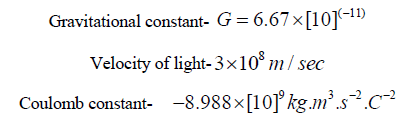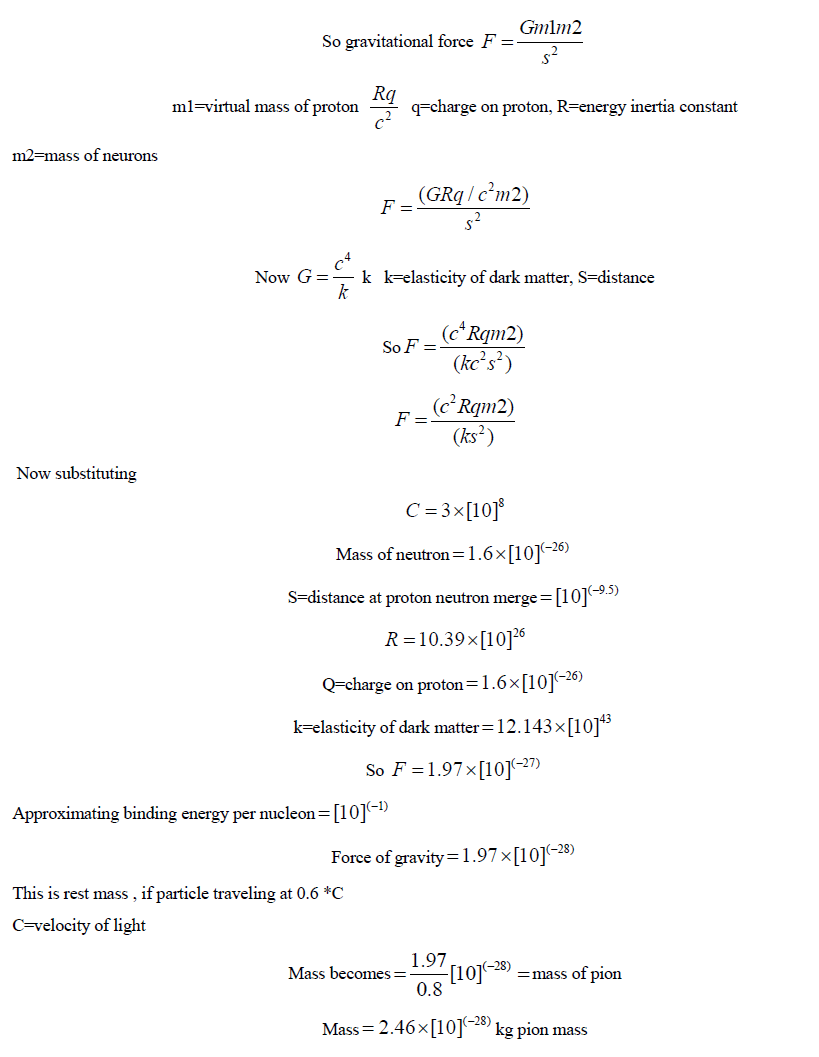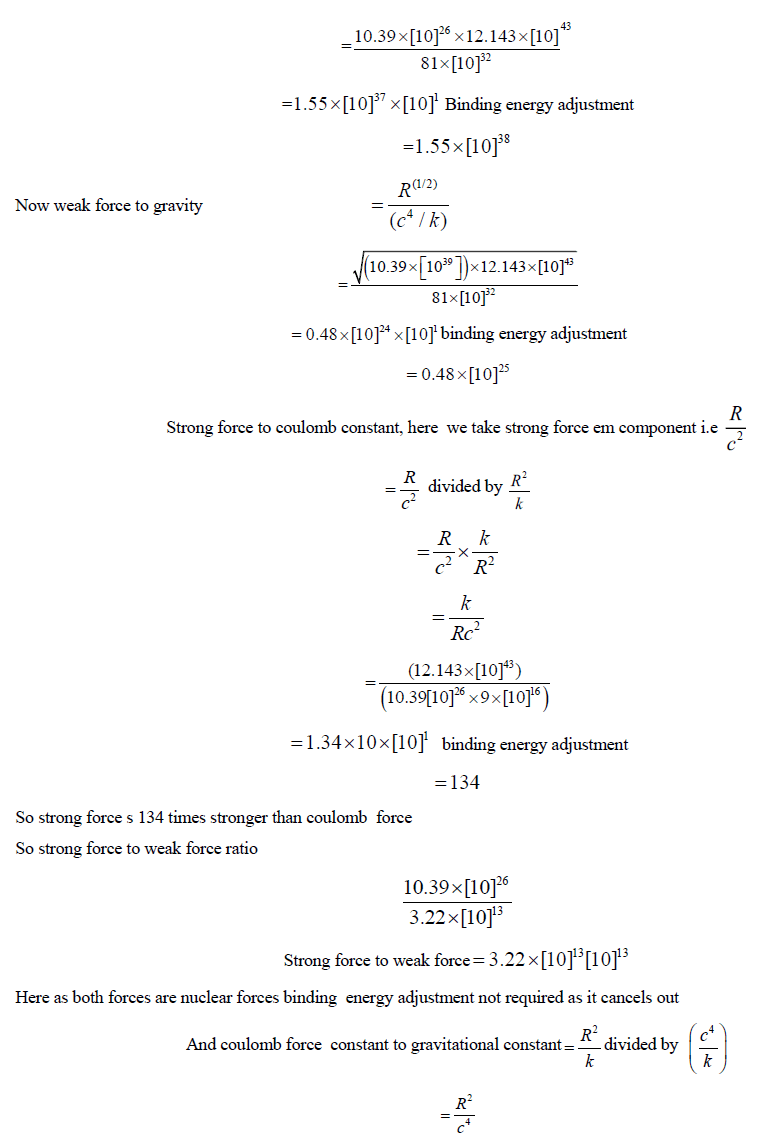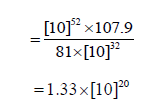Parası kalmadığı için otobüse binemiyordur ailesi porno izle ona daha yeni para gönderdiği için tekrar porno istemeye utanınca mecburen otostop çekmek için youporn çantasını alarak yol kenarına gelir etekli porno liseli türk kız yol kenarında dururken yanına yaklaşan porno kibar bir gencin onu gideceği yere kadar bırakmak porno izle istemesine çok mutlu olur arabaya bindiklerinde gideceği yer ile porno arabayı kullanan adamın gittiği yer arasında çok mesafe sex izle farkı olduğunu anlayan türk kız bu yaptığı porno indir iyilik karşısında arabada ona memelerini açar porno sapıklaşan adam yol kenarındaki hotelde durarak porno izle üniversiteli otostop çeken türk kızına odada sakso çektirip sikerExtending Concept of Dark Matter and Potential Energy acts Virtual Mass to Strong and Weak Force
All submissions of the EM system will be redirected to Online Manuscript Submission System. Authors are requested to submit articles directly to Online Manuscript Submission System of respective journal.

Viewpoint
, Volume: 11( 3) DOI: 10.37532/2319-9822.2021.11(3).205

# Extending Concept of Dark Matter and Potential Energy acts Virtual Mass to Strong and Weak Force

*Correspondence:
Shriprakash V PendseMumbai University, Mumbai, India; E-mail:[email protected]

Received:March 3, 2022, Manuscript No. tsse-21-32834; Published: March 27, 2022

Citation: Shriprakash V. Pendse. Extending Concept of Dark Matter and Potential Energy Acts Virtual Mass to Strong and Weak Force. J Space Explor. 2022;11(3):205.

### Abstract

Strong and weak equation can be derived through GR and energy mass equivalence -Exchange particle is nothing but gravitational force between particles, so force becomes particle. This is happening because confinement of per tube one particle. Mathematically we say weak and strong force acts through particle exchange. Weak force equation Gravitational constant and Q charge is square function. So weak force will create matter and antimatter with different charges and gravitational charges this solves strong cp problem as inertial constant is R linear function , it do not violate cp or cpt symmetry , only weak force violates CPT symmetry So inertial constants for weak=for strong=R In special relativity gives mass energy equivalence, GR with principal any energy field curves space time by gives mass charge equivalence principal but this mass is virtual mass And this virtual mass is dependent on geometry of area so for side of square With this model hierarchy problem can be solved Strong force same as EM force changes time arrow. Weak force creates two time arrows. So at Big bang T=0=1-1 two time arrows So except gravity all forces changes time arrow or creates two tie arrows. As clock wise fan which is perpetually in motion without friction in vacuum with zero gravity to move anticlockwise you require energy same is true about time arrow, as gravity is weak it can not do so, But in singularity gravity also changes time arrow. As I shown in first paper -K creates uncertainty principal, Yukwa model is achieved but weak force equation creates mathematical probability of –k or different gravitational fields. 16 Strong force inertial equation is , and weak force inertial equation is

### Keywords

Pion and W Boson, Gravitational Force, Inertial Weak Equation, Square Function, Weak Force Antimatter, Gravitational Field, Strong CP

### Introduction

Pion and w bosons are gravitational force between proton neutron in square area and side.

### Objective

• To find out inertial equation for weak and strong force
• To check force ratios
• To find out exchange particle mass
• ### Method used

law of gravity, energy mass equivalence, force as particle concept, quantum tubes are square shaped.

Data source

Data used is constants as per standard :Assumptions

• In getting weak and strong force calculation of gravitational force between proton and neutron where proton positive charge creates virtual mass.
• Proton neutron oscillates and merges to create pion at 10 -9.5 and separate at 10 (-15)neutron proton
• Proton and neutron merge where unbalanced neutron is a 10(-13.5) to create w-boson through side of square field and separate at 10(-18) as beta decay
• This w boson does beta minus decay
• From mathematic view strong force exchange particle is pion and weak force particle is w boson
• As strong force overpowers coulomb repulsion of proton it acts on square area so weak force acts through edge of side strong force constant is R and weak force constant under root R and charge also acts through edge it is also under root q
• This is happening as space tube are square shape, there is confinement per tube one particle
• So beta decay happens to conserve square symmetry so particle becomes stable
• Ladder shape atom

As in my first paper I shown potential energy can create virtual mass force of gravity between proton and neutron will be
equal to mass of neutron and virtual mass of proton FIG 1.So quantum level force becomes particle

For weak force force acts on side of square field. As strong force overpowers coulomb force it acts on on entire square field. So weak force acts on sides , so only change I strong force equation is R(1/2) and q(1/2)decay into pair , with different gravitational fields and electrical field .This solves all hierarchy problem in physics. Minor difference in nuclear forces is coming due to binding energy Force becomes particle: As I said in first paper quantum level discrete tubes structure postulated. So in tube only one particle can stay hence force becomes particle. And tubes are square shaped .

### Results

• Pion and w Boson are gravitational force between proton and neutron in square area and side
• Inertial weak equation shows gravity is square function
• Weak force will create matter antimatter with different gravitational field and charge field.
• Strong cp problem is solved as inertial constant of linear order while weak force constant is under root function. Strong force will never violate cp or cpt symmetry
• Strong force is super strong gravity , weak force is strong gravity, weak force can be attractive or repulsive, strong force is attractive
• ### Conclusions

• Strong and weak equation can be derived through GR and energy mass equivalence
• Exchange particle is nothing but gravitational force between particles, so force becomes particle.
• This is happening because confinement of per tube one particle.
• Mathematically we say weak and strong force acts through particle exchange.
• Weak force equation Gravitational constant and Q charge is square function.
• So weak force will create matter and antimatter with different charges and gravitational charges
• this solves strong cp problem as inertial constant is R linear function , it do not violate cp or cpt symmetry , only weak force violates CPT symmetry
•### REFERNCES

1. Shelden Glassho. Standard Model, particle exchange. Inference.2018;4
2. Steven Weinberg. The Making of the Standard Model. CERN. 2003.
3. Abdus Salam. Electroweak theory. Britannica Sci. 1960.
4. Albert Einstein. General Relativity, first effort to integrate gravity and electromagnetism. The berlin years. 1914;6.
5. Robort Hook. Hooks Law of elasticity. 1600.

###### Citations : 204

Journal of Space Exploration received 204 citations as per Google Scholar report

#### Indexed In

• Index Copernicus
• Open J Gate
• China National Knowledge Infrastructure (CNKI)
• Cosmos IF
• Directory of Research Journal Indexing (DRJI)
• Secret Search Engine Labs